## Example Questions

2 Next →

### Example Question #2 : How To Find Out If Lines Are Perpendicular

Which of the following lines is perpendicular to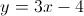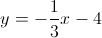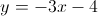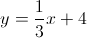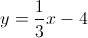Explanation:

The line which is perpendicular has a slope which is the negative inverse of the slope of the original line.

### Example Question #11 : New Sat Math No Calculator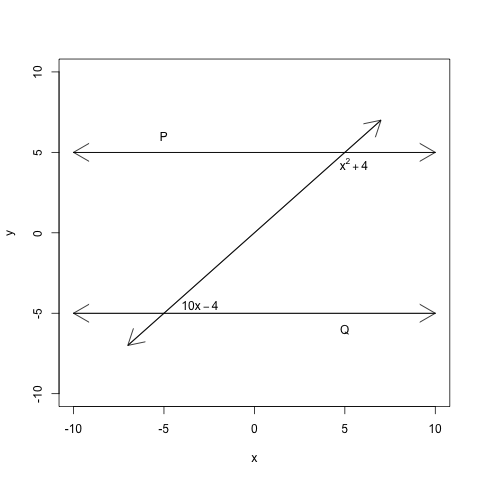Lines P and Q are parallel. Find the value of.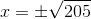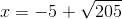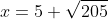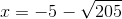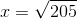Explanation:

Since these are complementary angles, we can set up the following equation.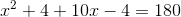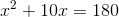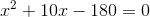Now we will use the quadratic formula to solve for.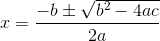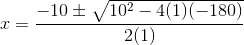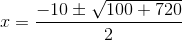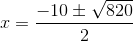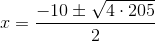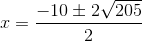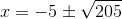Note, however, that the measure of an angle cannot be negative, sois not a viable answer. The correct answer, then, is2 Next →

### All SAT Math Resources